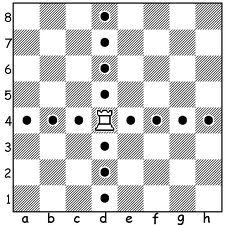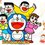# This note has been used to help create the Rook Polynomial wikiHello! After reading so many useful posts on Brilliant, I have finally set my mind to do one (my first!) on Rook's Polynomial, a useful technique for many combinatorics question (those with certain restrictions as you will see below).

To elaborate further on what exactly is the Rook's Polynomial, it is the generating function for the numbers of arrangement of $k$ non-attacking rooks on a board $B$. For those who are new to chess, rooks are chess piece that move and attack horizontally and vertically. Hence, for rooks to be non-attacking to each other, they must not be placed on the same row or column of the board.

In general, the rook polynomial of a $m \times n$ board $B_{m,n}$ (denoted as $R(x, B_{m,n})$) is: $R(x,B_{m,n}) = \displaystyle \sum_{k=0}^\infty r_k(B_{m,n})x^k$

where, $r_k$ is the number of ways to arrange $k$ non attacking rooks on board $B_{m,n}$. For example $R(x, B_{1,1}) = x +1$ since there are $1$ way (coefficient of $x^0 = 1$) to place $0$ non-attacking rook and $1$ way (coefficient of $x^1$) to place $1$ non-attacking rook.

So, how do we deduce a generalisation for the Rook's polynomial for $2$ -Dimensional board $B_{m,n}$? Letting $i = \min \{m,n\}$, we get $R(x,B_{m,n}) = \displaystyle \sum_{k=0}^i \binom{m}{k} P(n,k) \text{ } x^k$

The above can be derived by first choosing $k$ rows out of the board's $m$ rows; then permutating the $k$ out of the $n$ columns (denoted by $P(n,k)$). Recall that $k$ refers to the numbers of non attacking rooks placed on the board.

One way we can apply the Rook's Polynomial to combinatorics question is by labelling values/variables to the rows and columns of the board. A simple example question is as follows:

1. David, Mark, Jerry and Kai joined a card game club. They are required to choose $1$ out of the $5$ card games available in the club ---- namely, Monopoly Deal, Poker, Blackjacks, Uno and Cheat. How many ways are there for each of David, Mark, Jerry and Kai to choose a different card game from each other?

Solution: Label the rows of board $B_{4 \times 5}$ with the names (David, Mark, Jerry and Kai), and the columns with the card games (Monopoly Deal, Poker, Blackjacks, Uno and Cheat). Since each of the members must choose only $1$ card game and each card game can only be chosen by at most $1$ member, we can use the rook polynomial to find the ways to arranging $4$ non-attacking rooks on board $B_{4 \times 5}$. The answer to the question is hence, $\binom{4}{4} P(5,4) = 120$

Rook's Polynomial is a really interesting and (for me) quite a rare topic. Hope you have enjoyed and learnt something! Since there are much more about Rook's Polynomial, you can read it up here! :) Thanks!

• After you have read the above link: You can try this problem which can be solved by Rook's Polynomial.Note by Happy Melodies
6 years, 11 months ago

This discussion board is a place to discuss our Daily Challenges and the math and science related to those challenges. Explanations are more than just a solution — they should explain the steps and thinking strategies that you used to obtain the solution. Comments should further the discussion of math and science.

When posting on Brilliant:

• Use the emojis to react to an explanation, whether you're congratulating a job well done , or just really confused .
• Ask specific questions about the challenge or the steps in somebody's explanation. Well-posed questions can add a lot to the discussion, but posting "I don't understand!" doesn't help anyone.
• Try to contribute something new to the discussion, whether it is an extension, generalization or other idea related to the challenge.

MarkdownAppears as
*italics* or _italics_ italics
**bold** or __bold__ bold
- bulleted- list
• bulleted
• list
1. numbered2. list
1. numbered
2. list
Note: you must add a full line of space before and after lists for them to show up correctly
paragraph 1paragraph 2

paragraph 1

paragraph 2

[example link](https://brilliant.org)example link
> This is a quote
This is a quote
    # I indented these lines
# 4 spaces, and now they show
# up as a code block.

print "hello world"
# I indented these lines
# 4 spaces, and now they show
# up as a code block.

print "hello world"
MathAppears as
Remember to wrap math in $$ ... $$ or $ ... $ to ensure proper formatting.
2 \times 3 $2 \times 3$
2^{34} $2^{34}$
a_{i-1} $a_{i-1}$
\frac{2}{3} $\frac{2}{3}$
\sqrt{2} $\sqrt{2}$
\sum_{i=1}^3 $\sum_{i=1}^3$
\sin \theta $\sin \theta$
\boxed{123} $\boxed{123}$

Sort by:

Wow, I have never heard of this concept before. Thank you for sharing, very nice first post!

- 6 years, 11 months ago

Thanks for the encouragement :) have just read about it not long ago too

- 6 years, 11 months ago

Really brilliant, I've never seen such a good way of translating such ugly combinatorics questions into something with a fairly nice solution

- 6 years, 11 months ago

Nice explanation :) Thanks for the sample problems too!

- 6 years, 11 months ago

Thanks! :) the link above has more details and sample questions for you to explore!

- 6 years, 11 months ago

Superb.

- 6 years, 10 months ago

Very nice first post!

- 6 years, 10 months ago

Thanks!

- 6 years, 10 months ago

The link is also very informative....totally worth checking out

- 6 years, 10 months ago